# HSSlive: Plus One & Plus Two Notes & Solutions for Kerala State Board

## BSEB Class 10 Maths Chapter 9 Some Applications of Trigonometry Ex 9.1 Textbook Solutions PDF: Download Bihar Board STD 10th Maths Chapter 9 Some Applications of Trigonometry Ex 9.1 Book AnswersBSEB Class 10 Maths Chapter 9 Some Applications of Trigonometry Ex 9.1 Textbook Solutions PDF: Download Bihar Board STD 10th Maths Chapter 9 Some Applications of Trigonometry Ex 9.1 Book Answers

BSEB Class 10th Maths Chapter 9 Some Applications of Trigonometry Ex 9.1 Textbooks Solutions and answers for students are now available in pdf format. Bihar Board Class 10th Maths Chapter 9 Some Applications of Trigonometry Ex 9.1 Book answers and solutions are one of the most important study materials for any student. The Bihar Board Class 10th Maths Chapter 9 Some Applications of Trigonometry Ex 9.1 books are published by the Bihar Board Publishers. These Bihar Board Class 10th Maths Chapter 9 Some Applications of Trigonometry Ex 9.1 textbooks are prepared by a group of expert faculty members. Students can download these BSEB STD 10th Maths Chapter 9 Some Applications of Trigonometry Ex 9.1 book solutions pdf online from this page.

## Bihar Board Class 10th Maths Chapter 9 Some Applications of Trigonometry Ex 9.1 Books Solutions

 Board BSEB Materials Textbook Solutions/Guide Format DOC/PDF Class 10th Subject Maths Chapter 9 Some Applications of Trigonometry Ex 9.1 Chapters All Provider Hsslive

## How to download Bihar Board Class 10th Maths Chapter 9 Some Applications of Trigonometry Ex 9.1 Textbook Solutions Answers PDF Online?

2. Click on the Bihar Board Class 10th Maths Chapter 9 Some Applications of Trigonometry Ex 9.1 Answers.
3. Look for your Bihar Board STD 10th Maths Chapter 9 Some Applications of Trigonometry Ex 9.1 Textbooks PDF.
4. Now download or read the Bihar Board Class 10th Maths Chapter 9 Some Applications of Trigonometry Ex 9.1 Textbook Solutions for PDF Free.

## BSEB Class 10th Maths Chapter 9 Some Applications of Trigonometry Ex 9.1 Textbooks Solutions with Answer PDF Download

Find below the list of all BSEB Class 10th Maths Chapter 9 Some Applications of Trigonometry Ex 9.1 Textbook Solutions for PDF’s for you to download and prepare for the upcoming exams:

## BSEB Bihar Board Class 10th Maths Solutions Chapter 9 Some Applications of Trigonometry Ex 9.1

Question 1.
A circus artist is climbing a 20 m long rope, which is tightly stretched and tied from the top of a vertical pole to the ground. Find the height of the pole, if the angle made by the rope with the ground level is 30° (see figure).Solution:
In rt ∆ ABC, 𝐴𝐵𝐴𝐶 = sin 30°
So, 𝐴𝐵20 = 12
or AB = 12 x 20 m = 10 m
∴ Height of the pole is 10 m.

Question 2.
A tree breaks due to storm and the broken part bends so that the top of the tree touches the ground making an angle 30° with it. The distance between the foot of the tree to the point where the top touches the ground is 8 m. Find the height of the tree.
Solution: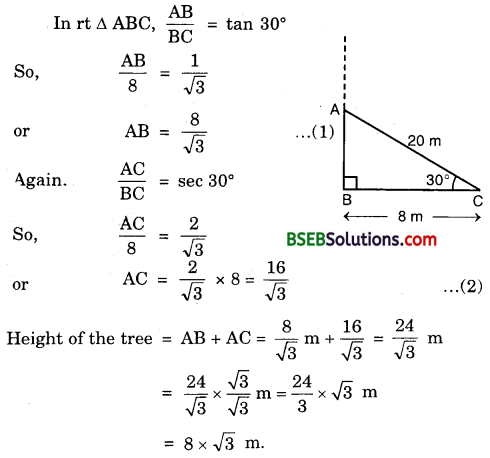Question 3.
A contractor plans to install two slides for the children to play in a park. For the children below the age of 5 years, she prefers to have a slide whose top is at a height of 1.5 m, and is inclined at an angle of 30° to the ground, whereas for elder children, she wants to have a steep slide at a height of 3 m, and inclined at an angle of 60° to the ground. What should be the length of the slide in each case?
Solution: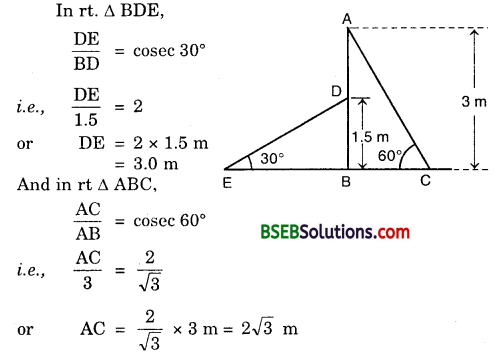∴ Length of the two slides are 3 m and 23‾√ m respectively.

Question 4.
The angle of elevation of the top of a tower from a point on the ground, which is 30 m away from the foot of the tower, is 30°. Find the height of the tower.
Solution:
Let AB be the tower of height h metres and let C be a point at a distance of 30 m from the foot of the tower. The angle of elevation of the top of the tower from point C is given as 30°.In ∆ CAB, we have :
𝐴𝐵𝐶𝐴 = tan 30°. So, ℎ30 = 13√
or h = 303√ = 103‾√
Hence, the height of the tower is 10-73 metres.

Question 5.
A kite is flying at a height of 60 m above the ground. The string attached to the kite is temporarily tied to a point on the ground. The inclination of the string with the ground is 60°. Find the length of the string, assuming that there is no slack in the string.
Solution:
Let OA be the horizontal ground, and let K be the position of the kite at height 60 m above the ground. Let the length of the string OK be x metres. It is given that ∠KOA = 60°.Hence, the length of the string is 403‾√ m.

Question 6.
A 1.5 m tall boy is standing at some distance from a 30 m tall building. The angle of elevation from his eyes to the top of the building increases from 30° to 60° as he walks towards the building. Find the distance he walked towards the building.
Solution:
Let OA be the building and PL’be the initial position of the boy such that ∠APR = 30° and AO = 30 m. Let MQ be the position of the boy after walking a distance PQ. Here, ∠AQR = 60°.
Now from ∆s ARQ and ARP, we have :Hence, the distance walked by the boy towards the building is 193‾√ metres.

Question 7.
From a point on the ground the angles of elevation of the bottom and top of a transmission tower fixed at the top of a 20 m high building are 45° and 60° respectively. Find the height of the tower.
Solution:
Let BC be the building of height 20 m and CD be the tower of height x metres.
Let A be a point on the ground at a distance of y metres away from the foot B of the building.
In ∆ ABC, we have :Hence, the height of the tower is 14.64 metres.

Question 8.
A statue 1.6 m tall stands on the top of a pedestal. From a point on the ground, the angle of elevation of the top of the statue is 60° and from the same point, the angle of elevation of the top of the pedestal is 45°. Find the height of the pedestal.
Solution:
Let BC be the pedestal of height h metres and CD be the statue of height 1.6 m.
Let A be a point on the ground such that ∠CAB = 45° and ∠DAB = 60°.
From ∆s ABC and ABD, we have :
𝐴𝐵𝐵𝐶 = cot 45°. So, 𝐴𝐵ℎ = 1
or AB = h … (1)
𝐵𝑑𝐴𝐵 = tan 60°. So, 𝐵𝐶+𝐶𝐷𝐴𝐵 = 3‾√
or ℎ+1.6ℎ = 3‾√ or h + 1.6 = h3‾√
or h(3‾√ – 1) = 1.60.8(3‾√ + 1) = 0.8(1.732 + 1) m = 2.19 m.

Question 9.
The angle of elevation of the top of the building from the foot of the tower is 30° and the angle of elevation of the top of the tower from the foot of the building is 60°. If the tower is 50 m high, find the height of the building.
Solution:
Let AB be the building of height h metres and AC, the horizontal ground through C, the foot of the tower. Since the building subtends an angle of 30° at C, hence ∠ACB = 30°. Let CD be the tower of 50 m height 50 m such that ∠CAD = 60°.From ∆s BAC and DCA, we have :
So, 𝐴𝐶ℎ = 3‾√
or AC = 3‾√ … (1)
and, 𝐷𝐶𝐴𝐶 = tan 60°. So, 50𝐴𝐶 = 3‾√
or AC = 503√
or AC = 503√ … (2)
Equating the values of AC from (1) and (2), we get
3‾√h = 503√
or h = 503√ x 13√ = 503 = 1623
Hence, the height of the building is 1623m.

Question 10.
Two poles of equal heights are standing opposite each other on either side of the road, which is 80 m wide. From a point between them on the road, the angles of elevation of the top of the poles are 60° and 30° respectively. Find the height of the poles and the distances of the point from the poles.
Solution:
Let AB and CD be two poles, each of height h metres. Let P be a point on the road such that AP = x metres. Then, CP = (80 – x) metres. It is given that
∠APB = 60° and ∠CPD = 30°.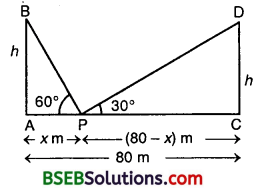In ∆ APB, we have :
𝐴𝐵𝐴𝑃 = tan 60°. So, ℎ𝑥 = 3‾√
or h = 3‾√ x
In ∆ CPD, we have :
𝐶𝐷𝐶𝑃 = tan 30°. So, ℎ80−𝑥 = 13√
or h = 80−𝑥3√
Equating the values of h from (1) and (2), we get
3‾√x = 80−𝑥3√ or 3x = 80 – x
So, 4x = 80 or x = 20
Putting x = 20 in (1), we get
h = 3‾√ x 20 = (1.732) x 20 = 34.64
Thus, the height of the pole is 34.64 metres. Further, the required point is at a distance of 20 metres from the first pole and 60 metres from the second pole.

Question 11.
A T.V. tower stands vertically on a bank of a river. From a point on the other bank directly opposite the tower, the angle of elevation of the top of the tower is 60°. From a point 20 m away from this point on the same bank, the angle of elevation of the top of the tower is 30° (See figure). Find the height of the tower and the width of the river.
Solution:
Let AB be the T.V. tower of height h metres standing on the bank of a river. Let C be the point on the opposite bank of the river such that BC = x metres. Let D be another point away from C such that CD = 20 m, ancLthe angles of elevation of the top of the T.V. tower from C and D are 60° and 30° respectively, i.e., ∠ACB = 60° and ∠ADB = 30°.
In ∆ ABC, we have :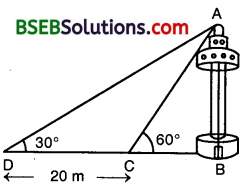Equating values of h from (1) and (2), we get
3‾√x + 𝑥+203√ or 3x = x + 20
or 3x – x = 20 or 2x = 20 or x = 10
Putting x = 10 in (1), we get
h = 3‾√ x 10 = (1.732) x 10 = 17.32
Thus, the height of the T.V. tower is 17.32 metres and the width of the river is 10 metres.

Question 12.
From the top of a 7 m high building, the angle of elevation of the top of a cable tower is 60° and the angle of depression of its foot is 45°. Determine the height of the tower.
Solution:
Let AB be the building of height 7 metres and let CD be the cable tower. It is given that the angle of elevation of the top D of the tower observed from A is 60° and the angle of depression of the base G of the tower observed from A is 45°.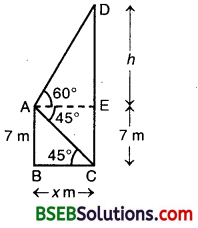Then, ∠EAD = 60° arid ∠EAC = 45°. Therefore,
∠BCA = ∠EAC = 45°.
Also, AB = 7 m.
In ∆ EAD, we have :
𝐷𝐸𝐸𝐴 = tan 60°
So, ℎ𝑥 = 3‾√
or h = 3‾√x … (1)
In ∆ ABC, we have :
𝐴𝐵𝐵𝐶 = tan 45°. So, 7𝑥 = 1
or x = 7
Putting x = 7 in (1), we get
h = 73‾√
So, DE = 73‾√ m
∴ CE + ED = (7 + 7 3‾√) m
= 7(3‾√ + 1) m = 7(1.732 + 1) m
= 7 x 2.732 m = 19.124 m
Hence, the height of the cable tower is 19.124 m.

Question 13.
As observed from the top of a 75 m tall lighthouse, the angles of depression of two ships are 30° and 45°. If one ship is exactly behind the other on the same side of the lighthouse, find the distance between the two ships.
[Annual Paper (Outside Delhi) 2008]
Solution:
Let AB be the lighthouse of height 75 m and let two ships be at C and D such that their angles of depression B are 45° and 30° respectively.
Let AC = x and CD = y.In ∆ ABC, we have :
𝐴𝐵𝐴𝐶 = tan 45°.
So, 75𝑥 = 1
or x = 75 … (1)
In ∆ ABD, we have :
𝐴𝐵𝐴𝐷 = tan 30°.
So, 75𝑥+𝑦 = 13√
or x + y = 753‾√ … (2)
From (1) and (2), we have :
75 + y = 753‾√ or y = 75(3‾√ – 1)
or y = 75(1.732 – 1) = 75 x 0.732 = 54.9
Hence, the distance between the two ships is 54.9 metres.

Question 14.
A 1.2 m tall girl spots a balloon moving with the wind in a horizontal line at a height of 88.2 m from the ground. The angle of elevation of the balloon from the eyes of the girl at any instant is 60°. After some time, the angle of elevation reduces to 30°. Find the distance travelled by the balloon during the interval.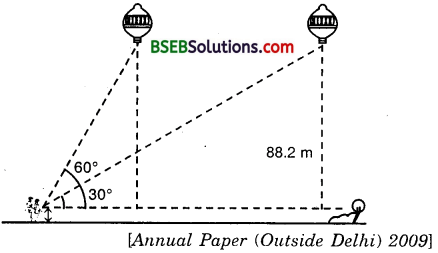Solution:
Let P and Q be the two positions of the balloon and let A be the point of observation. Let ABC be the horizontal through A. It is given that angles of elevation of the balloon in two positions P and Q from A are ∠PAB = 60° and ∠QAB = 30°. It is also given that
MQ = 88.2 m gives CQ = MQ – MC = (88.2 – 1.2) m = 87 metres.
In ∆ ABP, we have :In ∆ ACQ, we have :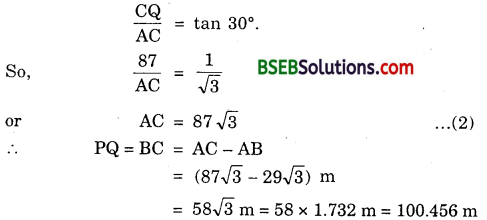Thus, the balloon travels 100.46 metres during the given interval.

Question 15.
A straight highway leads to the foot of a tower. A man standing at the top of the tower observes a car at an angle of depression of 30°, which is approaching the foot of the tower with a uniform speed. Six minutes later, the angle of depression of the car is found to be 60°. Find the time taken by the car to reach the foot of the tower.
Solution:
Let AB be the tower of height h. Let C be the initial position of the car and let after 6 minutes, the car be at D. It is given that the angles of depression at C and D are 30° and 60° respectively. Let the speed of the car be v metres per minute. Then,CD = Distance travelled by the car in 6 minutes.
or CD = 6v metres [∵ Distance = Speed x Time]
. Let the car takes t minutes to reach the foot of the tower AB from D. Then,
DA = vt metres
In ∆ ABD, we have :
𝐴𝐵𝐴𝐷 = tan 60°
or ℎ𝑣𝑡 = 3‾√ vt …. (1)
In ∆ ABC, we have :
𝐴𝐵𝐴𝐶 = tan 30° or ℎ𝑣𝑡+6𝑣 = 13√
or 3‾√ h = vt + 6v
Substituting the value of h from (1) in (2),
we get 3‾√ x 3‾√ vt = vt + 6v
or 3t = t + 6
or 3t – t = 6 or 2t = 6 or t = 3
Thus, the car will reach the tower from D in 3 minutes.

Question 16.
The angle of elevation of the top of a tower from two points at a distance of 4 m and 9 m from the base of the tower and in the same straight line with it are complementary. Prove that the height of the tower is 6 m.
Solution:
Let AB be the tower. Let C and D be the two points at distances 9 m and 4 m respectively from the base of the tower. Then, AC = 9 m and AD = 4 m.
Let ∠ACB = 0 then ∠ADB = 90° – 0.
[∵ Angles are complementry]
Let h metres be the height of the tower AB.
From ∆ ACB, we have :
𝐴𝐵𝐴𝐶 = tan θ
or ℎ9 = tan θ … (1)From ∆ ADB, we have :
𝐴𝐵𝐴𝐷 = tan (90° – θ)
or ℎ4 = cot θ … (2)
From (1) and (2), we have : h h
ℎ9 x ℎ4 = tan θ x cot θ
or h² = 36 or h = 6
Hence, the height of the tower is 6 metres.

## Bihar Board Class 10th Maths Chapter 9 Some Applications of Trigonometry Ex 9.1 Textbooks for Exam Preparations

Bihar Board Class 10th Maths Chapter 9 Some Applications of Trigonometry Ex 9.1 Textbook Solutions can be of great help in your Bihar Board Class 10th Maths Chapter 9 Some Applications of Trigonometry Ex 9.1 exam preparation. The BSEB STD 10th Maths Chapter 9 Some Applications of Trigonometry Ex 9.1 Textbooks study material, used with the English medium textbooks, can help you complete the entire Class 10th Maths Chapter 9 Some Applications of Trigonometry Ex 9.1 Books State Board syllabus with maximum efficiency.

## FAQs Regarding Bihar Board Class 10th Maths Chapter 9 Some Applications of Trigonometry Ex 9.1 Textbook Solutions

#### How to get BSEB Class 10th Maths Chapter 9 Some Applications of Trigonometry Ex 9.1 Textbook Answers??

Students can download the Bihar Board Class 10 Maths Chapter 9 Some Applications of Trigonometry Ex 9.1 Answers PDF from the links provided above.

#### Can we get a Bihar Board Book PDF for all Classes?

Yes you can get Bihar Board Text Book PDF for all classes using the links provided in the above article.

## Important Terms

Bihar Board Class 10th Maths Chapter 9 Some Applications of Trigonometry Ex 9.1, BSEB Class 10th Maths Chapter 9 Some Applications of Trigonometry Ex 9.1 Textbooks, Bihar Board Class 10th Maths Chapter 9 Some Applications of Trigonometry Ex 9.1, Bihar Board Class 10th Maths Chapter 9 Some Applications of Trigonometry Ex 9.1 Textbook solutions, BSEB Class 10th Maths Chapter 9 Some Applications of Trigonometry Ex 9.1 Textbooks Solutions, Bihar Board STD 10th Maths Chapter 9 Some Applications of Trigonometry Ex 9.1, BSEB STD 10th Maths Chapter 9 Some Applications of Trigonometry Ex 9.1 Textbooks, Bihar Board STD 10th Maths Chapter 9 Some Applications of Trigonometry Ex 9.1, Bihar Board STD 10th Maths Chapter 9 Some Applications of Trigonometry Ex 9.1 Textbook solutions, BSEB STD 10th Maths Chapter 9 Some Applications of Trigonometry Ex 9.1 Textbooks Solutions,
Share: